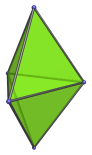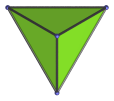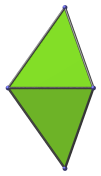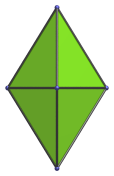# The Triangular Bipyramid

The triangular bipyramid is the 12th Johnson solid (J12). It has 5 vertices, 9 edges, and 6 faces (6 equilateral triangles).The triangular bipyramid can be constructed by attaching two tetrahedra to each other.

If a triangular prism is inserted between the two constituent tetrahedra, an elongated triangular bipyramid (J14) is produced.

The triangular pyramid (J12) is the dual of a non-uniform triangular prism, one with rectangular faces with edge ratio √6 : 1 rather than squares. The dual of the uniform triangular prism is a different triangular pyramid—one with isosceles triangles (with edge ratio 2 : 3) rather than equilateral triangles.

## Projections

Here are some views of the triangular bipyramid from various angles:

Projection Envelope DescriptionTriangle

Top view.Tetragon

Side view.Rhombus

Front view.

## Coordinates

The Cartesian coordinates of the triangular bipyramid with edge length 2 are:

• (±1, −1/√3, 0)
• (0, 2/√3, 0)
• (0, 0, ±√(8/3))

Last updated 10 Jan 2023.